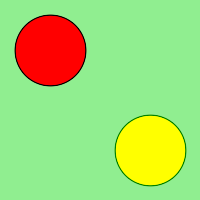# Wand push() and pop() in Python

We can use ImageMagick’s internal graphic context stack to manage different styles and operations in Wand. There are total four push functions for context stack.

• push()
• push_clip_path()
• push_defs()
• push_pattern()

push() function is used to grow context stack and pop() is another function and used to restore stack to previous push.

Syntax :

```# for push()
wand.drawing.push()

# for pop()
wand.drawing.pop()```

Parameters : No parameters for push() as well as for pop() function

Example #1:

## Python3

 `from` `wand.image ``import` `Image` `from` `wand.drawing ``import` `Drawing` `from` `wand.color ``import` `Color`   `with Drawing() as ctx:` `    ``ctx.fill_color ``=` `Color(``'RED'``)` `    ``ctx.stroke_color ``=` `Color(``'BLACK'``)` `    ``ctx.push()`   `    ``ctx.circle((``50``, ``50``), (``25``, ``25``))` `    ``ctx.pop()`   `    ``ctx.fill_color ``=` `Color(``'YELLOW'``)` `    ``ctx.stroke_color ``=` `Color(``'GREEN'``)` `    ``ctx.push()`   `    ``ctx.circle((``150``, ``150``), (``125``, ``125``))` `    ``ctx.pop()`   `    ``with Image(width ``=` `200``, height ``=` `200``, background ``=` `Color(``'lightgreen'``)) as image:` `        ``ctx(image)` `        ``image.save(filename ``=` `"push.png"``)`

Output:Example #2:

## Python3

 `from` `wand.color ``import` `Color` `from` `wand.image ``import` `Image` `from` `wand.drawing ``import` `Drawing` `from` `wand.compat ``import` `nested` `from` `math ``import` `cos, pi, sin`   `with nested(Color(``'lightblue'``),` `            ``Color(``'transparent'``),` `            ``Drawing()) as (bg, fg, draw):` `    ``draw.stroke_width ``=` `3` `    ``draw.fill_color ``=` `fg`   `    ``for` `degree ``in` `range``(``0``, ``360``, ``15``):` `        ``draw.push()  ``# Grow stack` `        ``draw.stroke_color ``=` `Color(``'hsl({0}%, 100 %, 50 %)'``.``format``(degree ``*` `100` `/` `360``))` `        ``t ``=` `degree ``/` `180.0` `*` `pi` `        ``x ``=` `35` `*` `cos(t) ``+` `50` `        ``y ``=` `35` `*` `sin(t) ``+` `50` `        ``draw.line((``50``, ``50``), (x, y))` `        ``draw.pop()  ``# Restore stack`   `    ``with Image(width ``=` `100``, height ``=` `100``, background ``=` `Color(``'green'``)) as img:` `        ``draw(img)` `        ``img.save(filename ``=` `"pushpop.png"``)`

Output:Whether you're preparing for your first job interview or aiming to upskill in this ever-evolving tech landscape, GeeksforGeeks Courses are your key to success. We provide top-quality content at affordable prices, all geared towards accelerating your growth in a time-bound manner. Join the millions we've already empowered, and we're here to do the same for you. Don't miss out - check it out now!

Previous
Next